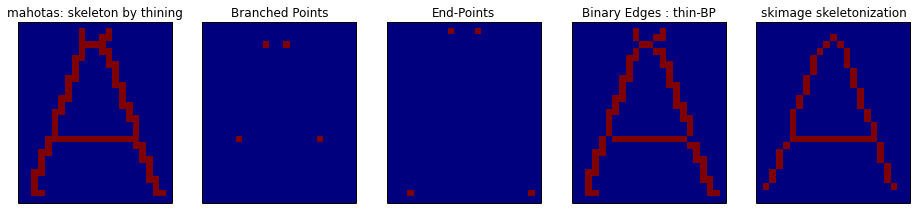## Friday, May 30, 2014

### Construct a graph from the skeleton image of a binary form

Previously an attempt was made to write a code capable of building a graph from the skeleton of an image and it was not a complete sucess since some preprocessing (pruning) had to be done to build a graph. Here an other python code was written and at very first sight, it seems to produce a graph without complaining....sometimes (see at the end).
The code was run in a ipython notebook running on sagemath cloud. It relies on several libraries : mahotas and networkx, numpy is provided by default. Scikit image was also tested. Pillow was used to generate images of some letter as model.

The idea is the following:
• make the skeleton of a binary image.
• get its branched-points and add them in a graph.
• get its end-points, add them to the graph.
• get the edges of the skeleton and decompose them into:
• edges between end-points and branched-points.
• edges between branched points.
• add edges to the graph between end-points and branched-points.
• add edges to the graph between branched-points.

## Images models

Since the code is run on sagemath cloud, Pillow has to be installed. From a terminal :
`pip install Pillow`
The verdana true type font was obtained from fontsupply and the file verdana.ttf had to be at the same location than the ipython notebook ( verdana.ttf was uploaded on SMC project at the same location than the ipython notebook).

The code used to generate images and draw them is:

and we get:

## What kind of skeleton?

Both mahotas an scikit-image implement morphological operators to generate the skeleton of a binary shape. Scikit-image seems to use a neighbourhood  on 4 pixels (C4) and mahotas on on neighbourhood of 8 pixels (C8). This is why mahotas was retained to produce skeletons:
• medial axis  of the letter A computed with scikit image (left), branched points and end-points are extracted using hit-or-miss operator implemented in mahotas (middle). The branched-points can be removed from the medial axis and the resulting image can be labelled (left), pixels connected only vertically or horizontally share the same label.Skeleton from the letter A (left)
•  If a skeleton is generated by morphological thining with mahotas, we get the left image. The fourth image is the skeleton deprived of its branched points. Scikit-image provide a thining operator with a C4 neighborhood (right image):C8 skeleton by thining (left). C4 skeleton by thining (right).

What's happen if a C8 skeleton is labeled? Here, both mahotas and scikit-image provide the same result:edges (mahotas:left; scikit-image:right)
The labelled regions fit here what can be seen as an edges.

## Prior to start to build a graph, several additionnal images are needed:

### Edges decomposition:

Let's decompose the image of edges by considering edges connecting end-points to branched-points and edges connecting only branched points:

### Starting to build the graph by the branched-points:

The branched-points are added first. The branched point of label 1 in the image, is the first vertex in the graph, and so on:

The end points are the added to the graph, there is a shift between the end-points label in the image and their index in the graph. The first end-point in the image will be the fith vertex in the graph:
The vertex of the graph (G) have attributes derived from the images they come from: their position 'pos' define by (row,col), their label in the image of origin. Typing in a ipython cell:
print G.nodes(data=True)
print nx.get_node_attributes(G,'label')
yields:
```[(1, {'pos': (3, 9), 'label': 1}), (2, {'pos': (3, 12), 'label': 2}), (3, {'pos': (17, 5), 'label': 3}), (4, {'pos': (17, 17), 'label': 4}), (5, {'pos': (1, 9), 'label': 1}), (6, {'pos': (1, 13), 'label': 2}), (7, {'pos': (25, 3), 'label': 6}), (8, {'pos': (25, 21), 'label': 8})]
{1: 1, 2: 2, 3: 3, 4: 4, 5: 1, 6: 2, 7: 6, 8: 8}
```

### Linking end-points to branched points:

The idea here is to get the neighbourhood of each branched-point in the "edges ep-ep" image and to add an edge in the graph between the corresponding branch-point and end-point(s). This yield:

•  A dictionnary where the keys are the labels of the branched-points and the values are the label of the "edges bp-bp" in the neighbourhood of the branched point is build. Thus there's one key (bp label) for several values (edges labels).
• But an edge can be seen as one key (the edge label) for two values (the two labels of the two branched-points). So the dictionnary has to be inverted in that very particular way. Fortunately, that problem was solved on stackoverflow.
Finaly, we have:
the whole steps are gathered in a function:

Processing other  letters gives for example:

## The ipython notebook is here:

View the notebook on ipython.org

## Sometimes building the graph fails:

On a larger set of images:
• For the image corresponding to the letter K, the graph construction fails due to the presence of branched regions made of several pixels instead of one.
• There's also a problem with the letter D, its graph has no loop.
• Since there's no branched point in the skeleton of the letter C, its graph is not build.
The ipython notebook has been reordered and is available on ipython.org